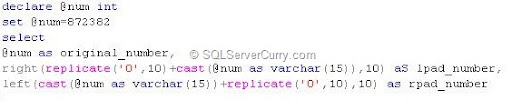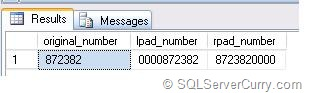Oracle has two formatting functions LPAD and RPAD which formats the number by appending leading and trailing zeros respectively. SQL Server does not have direct equivalent functions.

However you can simulate these functions using other string functions available in SQL Server. Let us take a practical example. Suppose you want to export data to fixed format file and the requirement is that the number should be 10 digits long, in such a way that if the total number of digits is less than 10, the remaining digits should be filled with zeroes.

Consider the following exampledeclare @num int
set @num=872382
select

The above code shows numbers in two formats. The first is left padded and second is right padded. The replicate function is used to replicate 0 for 10 times and the actual number is converted to string and then appended with zeroes.

The right function picks the last 10 digits from the result, so that it has 4 leading zeroes, as the original number shown in the code above has only 6 digits. We then reverse the same by converting a number to varchar and appending leading zeroes at the end. We then use the LEFT function which picks the number where last 4 digits are zeroes.

OUTPUT